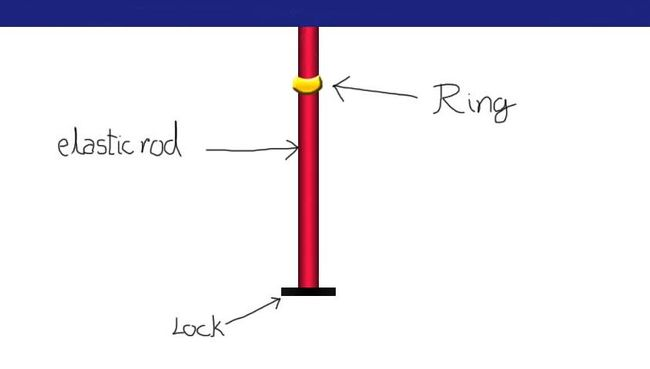# Elongation in rodAn elastic rod of costant $k$ and length $l$ is hung at a point P, rod have a massless lock at the other end. A smooth ring of mass $m$ falls from P (It is the same point from where the rod was hung), find the max elongation in the rod.

Details and Asumptions:

• Gravity acts vertically downwards.

• Position of ring in picture is not of initial position.

• Length l is taken from point P to lock.

• $l=30, m=20, k=10, g=10$

• All the values above are in SI units

×